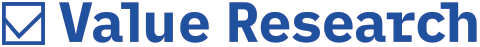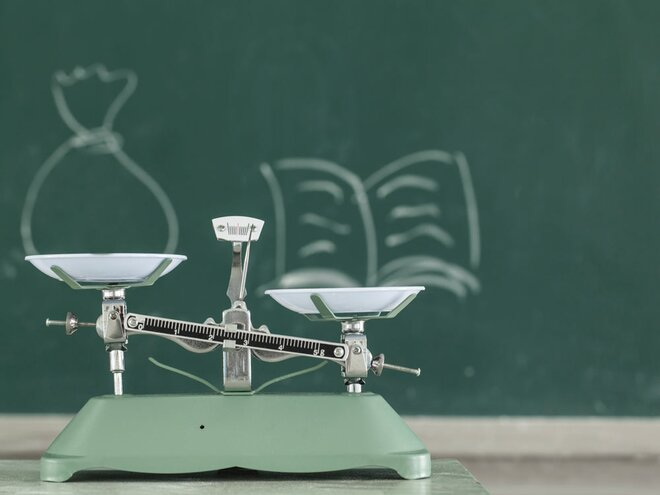# Understanding the P/B ratio

##### The P/B ratio is a classic tool to assess valuations, especially when the P/E ratio is not useful. Here is all that you need to know about itValuation metrics refer to the tools used to evaluate the financial strength of companies and are computed by making calculations using the data disclosed in the balance sheet, income statement and cash-flow statement. These ratios assess companies' profitability, liquidity, operational efficiency and stability, thereby providing investors with in-depth information on companies. By leveraging the power of ratio analysis, investors can make well-informed decisions.

The price-to-book ratio (P/B ratio) is a commonly used tool by value investors. Unlike the P/E ratio which mainly focuses on a company's earnings, the P/B ratio looks at how expensive a company is as compared to its assets (after paying off its liabilities). It is computed by dividing the price per share (or the company's market capitalisation) with the book value per share (or the company's net worth).

Let's illustrate this with the example of Reliance Industries, which is trading at around Rs 1995 per share and has a book value of Rs 736. So, its P/B ratio is 2.71. This means that investors are ready to pay Rs 2.71 for every rupee of net assets owned by Reliance Industries. If a company is trading at a P/B of less than one, this implies that investors can buy one rupee worth of assets for less than a rupee.

Advantages
Similar to the P/E ratio, the P/B ratio gives an easily understandable picture of the company's valuation in relation to its net worth. It is more stable (since asset prices are not as volatile as earnings) and can also be used to value companies which have not earned profits (start-ups and companies going through liquidation).

Disadvantages
However, this metric is based on the accounting recognition of assets and therefore, does not capture the true value of all the company's assets because the accounting system has not yet evolved to value intangible assets such as ideas, human capital, distribution networks and hard-to-value intellectual property. While book value might have been extremely relevant in the past when physical and tangible assets such as land, factory, equipment and buildings were the primary assets required for running a business, its relevance is decreasing in the modern era when the proportion of intangible assets is increasing(ex: e-commerce firms).

Therefore, the P/B ratio of companies having a greater proportion of intangible assets will seem optically higher than those having equal amounts of tangible assets.

Another drawback of this metric is that the value of assets is subject to management's discretion because entries like depreciation and goodwill can be manipulated to either increase or decrease the book value of assets.

Also, this metric cannot be applied to companies having negative net worth. For example, SpiceJet is currently having a negative net worth. And this could happen with startups as well as mature companies going through a difficult phase.

Also read in this series
Understanding the P/E ratio